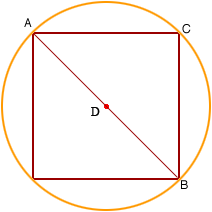Quandaries and Queries Who is asking: Other Level: Elementary Question: my granddaughter asked what is the largest size square in inches would fit in a 60 inch circle? I believe it to be around 42.3 inches but would like to teach her how to do it mathematically. bob Hi Bob, The argument requires the Pythagorean Theorem. Draw a circle with a square, as large as possible, inside the circle.By the symmetry of the diagram the center of the circle D is on the diagonal AB of the square. Hence AB is a diagonal of the circle and thus its length of is 60 inches and the lengths of BC and CA are equal. The Pythagorean Theorem then says that |BC|2 + |CA|2 = |AB|2 Hence 2 |BC|2 = 602 = 3600 and therefore |BC|2 = 1800. Taking the square root on my calculator I get |BC| = 42.43 Penny Go to Math Central